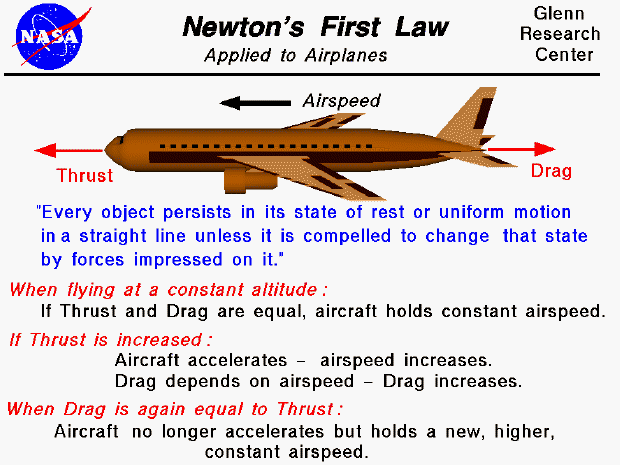Sir Isaac Newton first presented his three laws of motion in the "Principia Mathematica Philosophiae Naturalis" in 1686. His first law states that every object will remain at rest or in uniform motion in a straight line unless compelled to change its state by the action of an external force. This is normally taken as the definition of inertia. The key point here is that if there is no net force resulting from unbalanced forces acting on an object (if all the external forces cancel each other out), then the object will maintain a constant velocity. If that velocity is zero, then the object remains at rest. And if an additional external force is applied, the velocity will change because of the force.

An aircraft in flight is a particularly good example of the first law of motion. There are four major forces acting on an aircraft; lift, weight, thrust, and drag. If we consider the motion of an aircraft at a constant altitude, we can neglect the lift and weight. A cruising aircraft will fly at a constant airspeed and the thrust will exactly balance the drag of the aircraft. This is the first part sited in Newton's first law; there is no net force on the airplane and it travels at a constant velocity in a straight line.

Now if the pilot changes the thrust of the engine, the thrust and drag are no longer in balance. If the thrust is increased, the aircraft will accelerate and the velocity will increase. This is the second part sited in Newton's first law; an external force will change the velocity of the object. The drag of the aircraft, like the lift, depends on the square of the velocity. So the drag will increase with increased velocity. Eventually, the new drag will be equal to the new thrust level and at that point, the forces will again balance out, and the acceleration will stop. The airplane continues to fly at a new constant velocity that would be higher than the initial velocity. We are again back to the first part of the law with the aircraft traveling at a constant velocity.

Remember, in this simple example, that we are only considering the motion of the aircraft in a horizontal direction; we are neglecting any effects of the thrust on weight or on lift. On real aircraft, increasing the throttle setting increases the fuel usage and decreases the weight, and the increase in velocity will increase the lift as well as the drag. Each of these changes will affect the vertical motion of the aircraft, but to simplify our understanding of Newton's laws, we are neglecting these effects in this example.

It's important to point out the role of engine thrust in this example. Thrust is used to accelerate the aircraft, to change it's velocity, and thrust is used to balance the drag when the aircraft is cruising at a constant velocity. We normally think of only the first role - acceleration. For fighter planes high thrust is desirable. But some aircraft, like jet airliners, spend most of their existence in cruising flight balancing the drag. In that role engine efficiency (low fuel usage) is desirable. Depending on the mission of the aircraft, different types of engines are designed to provide high thrust or high efficiency.Guided Tours